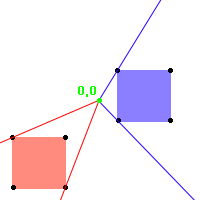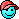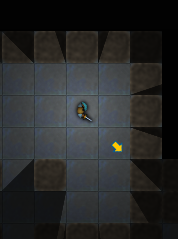A Flash Developer Resource Site

# Thread: How to find points "clockwise" relative to origin?

1. ## How to find points "clockwise" relative to origin?

I have a collection of points that defines a polygon. For example, let's say the blue square bounded by these points: 20 x -30, 70 x -30, 70 x 20, 20 x 20:I want to find the two points in the polygon that define its edges relative to an observer at the origin. That is, if you were standing at 0,0 which two points would you say were the most clockwise and the most counter-clockwise?

I'm having a hard time thinking of a clean solution that handles all cases, such as the blue square where some of its points have a negative angle relative to origin and some have a positive angle.

• This is for a shadow casting algorithm.
• The origin is not expected to fall within the polygon.Reply With Quote

2. Is it safe to assume the origin will be outside the polygon's convex hull?Reply With Quote

3. I think you can use the 2D cross product. Define it for 2 vectors A and B:

Code:
`cross2D(A,B) := A.x*B.y - B.x*A.y`
I'm assuming your coordinates are right handed, you might need to twiddle the sign if it's not.

You want to find the pair of points that maximizes the cross product (measured from the origin). In psuedocode:

Code:
```Number best_cross = 0.0;
Array best_vertex_pair;
for (a = first vertex to last)
for (b = first vertex to last)
if (a != b)
{
Number current_cross = cross2D(a,b);
if (current_cross > best_cross)
{ best_cross = current_cross; best_vertex_pair = [a,b]; }
}```
Upon completion, I think best_vertex_pair will have the info you want.

Hope it works!Reply With Quote

4. Edit, actually thats not right. When the best pair subtends and angle greater than 90 degrees I think it will break down. I will give it more thought.Reply With Quote

5. You pretty much got the answer I was thinking -- but you can search for the largest angle instead of cross-product. It should work as long as you aren't inside the polygon's convex hull (meaning it works for angles up to 180 degrees).To get it easily, calculate the absolute angle from the origin to each point.

Code:
```vert[];
angles[];
for(i = 0; i < vert.length; ++i)
angles[i] = Math.atan2(vert[i].y, vert[i].x);```
Then calculate the difference between each pair of absolute angles, being careful with overflows. The pair of points with the largest angleDiff is your answer.

Code:
```angleDiff(a, b) {
diff = abs(a-b);
if(diff > pi) return diff-pi;
return diff;
}```Reply With Quote

6. I gave it some more thought, and I think you can avoid the winding/overflow issues by minimizing the dot product over the pairs which have positive cross product. New pseudocode:

Code:
```Number smallest_dot = 1.0;
Array best_vertex_pair;
for (a = first vertex to last)
for (b = first vertex to last)
if (a != b && cross2D(a,b) > 0)
{
Number current_dot = dot2D( normalize(a), normalize(b) );
if (current_dot < smallest_dot)
{ smallest_dot = current_dot; best_vertex_pair = [a,b]; }
}```Reply With Quote

7. if your polygons are convex, the 2 vertices will each contribute to one edge whose normal points toward the origin and one whose normal points away from it. this assumes, as you've stated, that the vertices are defined relative to light's origin.
Code:
```extremeVertices = [];

for vertex in vertices
{

edge 1 = vertex - vertex.prev
edge 2 = vertex.next - vertex

edge normal 1 = perp( edge 1 )
edge nomral 2 = perp( edge 2 )

if( !areSameSign( dot( vertex, edge normal 1 ), dot( vertex, edge normal 2 ) ) )
{
extremeVertices.push( vertex )
}

}

Boolean areSameSign( Number n1, Number n2 ) { return n1 * n2 >= 0 }
Vector2D perp( Vector2D v ) { return Vector2D( -v.y, v.x ) }```
if a polygon isn't convex, you can use its convex hull for the shadow it casts NOT onto itself.Reply With Quote

8. I found the answer I was looking for with front-face culling based on the dot product. Which turned out not to be the answer I wanted because it looked strange when an occlusion shades a nearby object, but not itself:Note the triangle of shadow a wall casts on its neighbors looks bizarre because the wall itself is illuminated. I eliminated this by instead casting shadows from the front faces.

This added the new problem that although the lighting looked right, the walls were basically completely black. But I think I'll be able to produce a good compromise by rendering walls and other occluders on top of the shadow stencil with their luminosity based on surrounding squares.Reply With Quote

geometry, los, shadows, visibility####Posting Permissions

• You may not post new threads
• You may not post replies
• You may not post attachments
• You may not edit your posts
•

 » Home » Movies » Tutorials » Submissions » Board » Links » Reviews » Feedback » Gallery » Fonts » The Lounge » Sound Loops » Sound FX » About FK » Sitemap